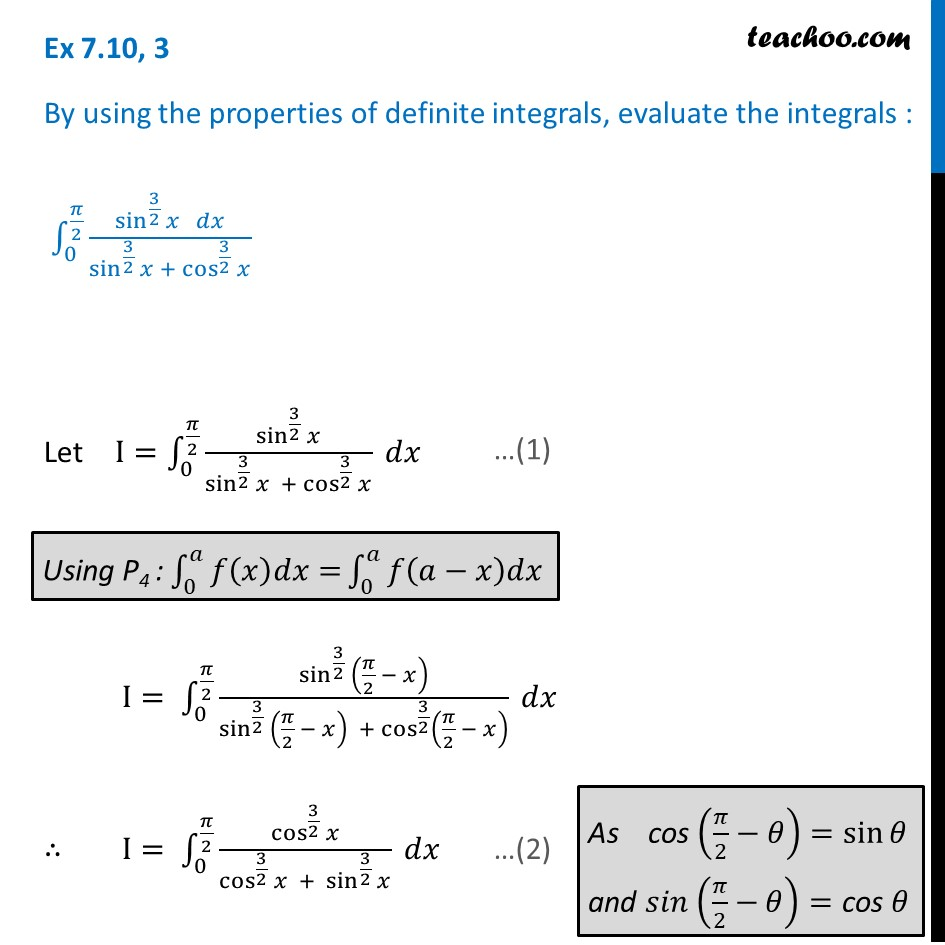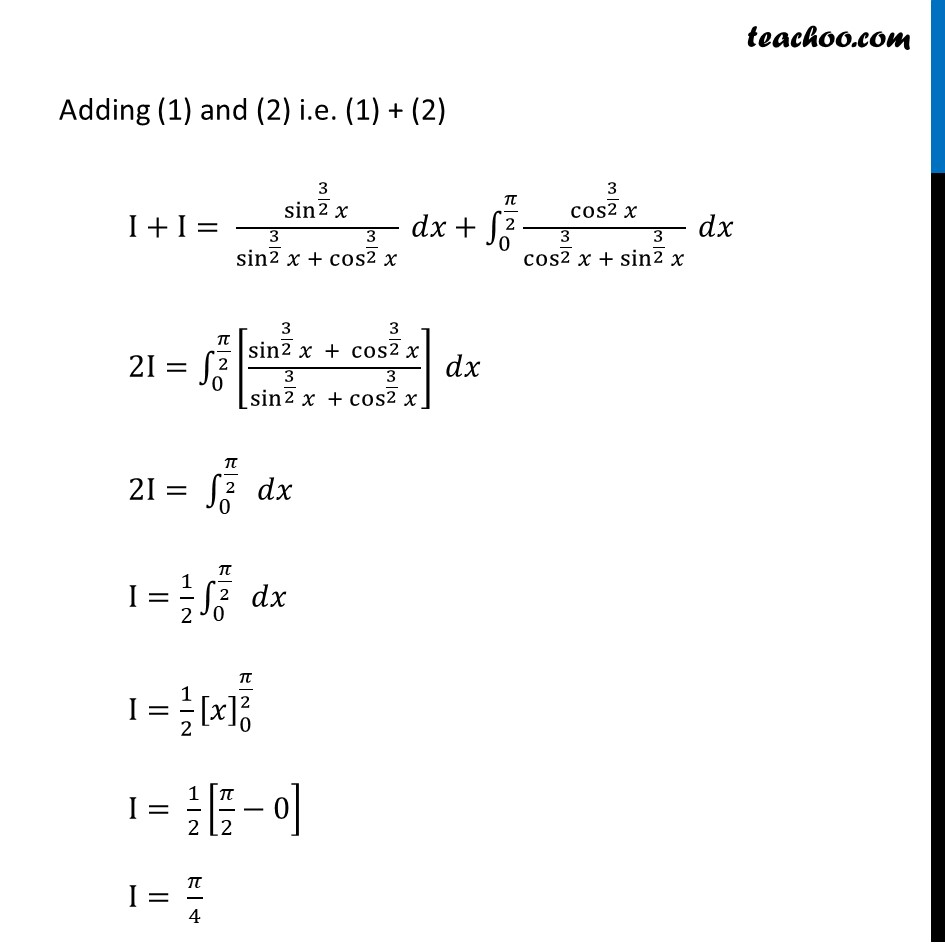Definite Integration by properties - P4

Chapter 7 Class 12 Integrals
Concept wiseLearn in your speed, with individual attention - Teachoo Maths 1-on-1 Class

### Transcript

Ex 7.10, 3 By using the properties of definite integrals, evaluate the integrals : ∫_0^(𝜋/2)▒(sin^(3/2)⁡𝑥 𝑑𝑥)/(sin^(3/2)⁡𝑥 + cos^(3/2)⁡𝑥 ) Let I=∫_0^(𝜋/2)▒〖(sin^(3/2) 𝑥)/(sin^(3/2) 𝑥 + cos^(3/2)⁡𝑥 ) 𝑑𝑥〗 I= ∫_0^(𝜋/2)▒〖(sin^(3/2) (𝜋/2 − 𝑥))/(sin^(3/2) (𝜋/2 − 𝑥) + cos^(3/2) (𝜋/2 − 𝑥) ) 𝑑𝑥〗 ∴ I= ∫_0^(𝜋/2)▒〖cos^(3/2)⁡𝑥/(cos^(3/2)⁡𝑥 + sin^(3/2)⁡𝑥 ) 𝑑𝑥〗 Adding (1) and (2) i.e. (1) + (2) I+I= sin^(3/2)⁡𝑥/(sin^(3/2)⁡𝑥 + cos^(3/2)⁡𝑥 ) 𝑑𝑥+∫_0^(𝜋/2)▒〖cos^(3/2)⁡𝑥/(cos^(3/2)⁡𝑥 + sin^(3/2)⁡𝑥 ) 𝑑𝑥〗 2I=∫_0^(𝜋/2)▒〖[(sin^(3/2)⁡𝑥 + cos^(3/2)⁡𝑥)/(sin^(3/2)⁡𝑥 + cos^(3/2)⁡𝑥 )] 𝑑𝑥〗 2I= ∫_0^(𝜋/2)▒〖 𝑑𝑥〗 I=1/2 ∫_0^(𝜋/2)▒〖 𝑑𝑥〗 I=1/2 [𝑥]_0^(𝜋/2) I= 1/2 [𝜋/2−0] I= 𝜋/4